# PSAT Math : How to find the volume of a tetrahedron

## Example Questions

### Example Question #2 : Tetrahedrons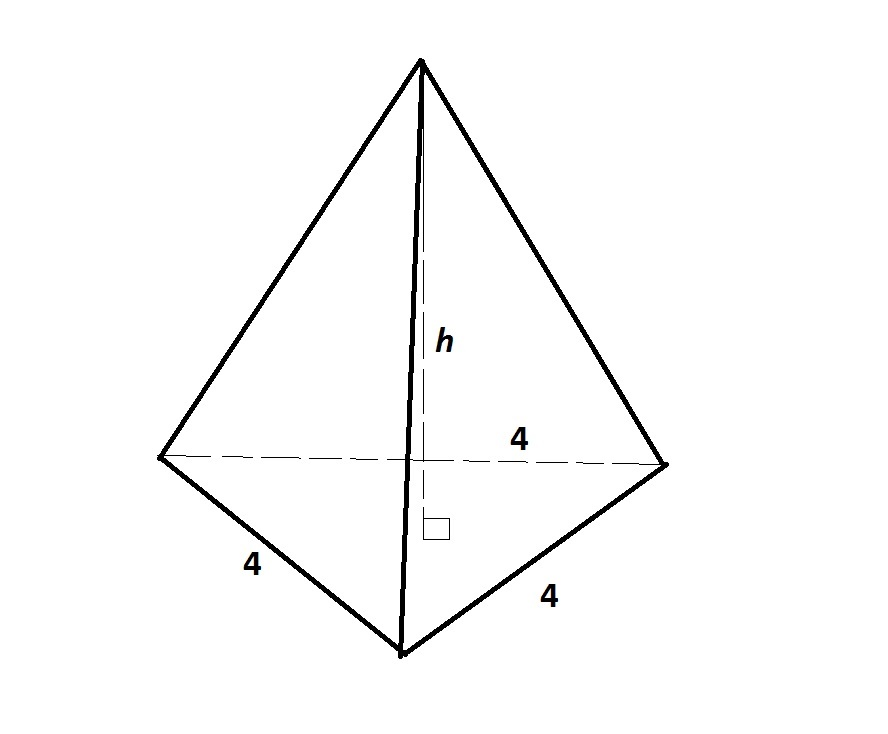Note: Figure NOT drawn to scale.

The above triangular pyramid has volume 25. To the nearest tenth, evaluate.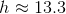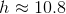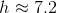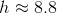Insufficient information is given to answer the problem.Explanation:

We are looking for the height of the pyramid.

The base is an equilateral triangle with sidelength 4, so its area can be calculated as follows: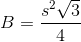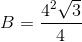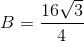The heightof a pyramid can be calculated using the fomula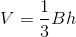We set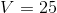and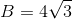and solve for: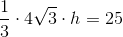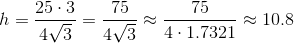### Example Question #81 : Solid Geometry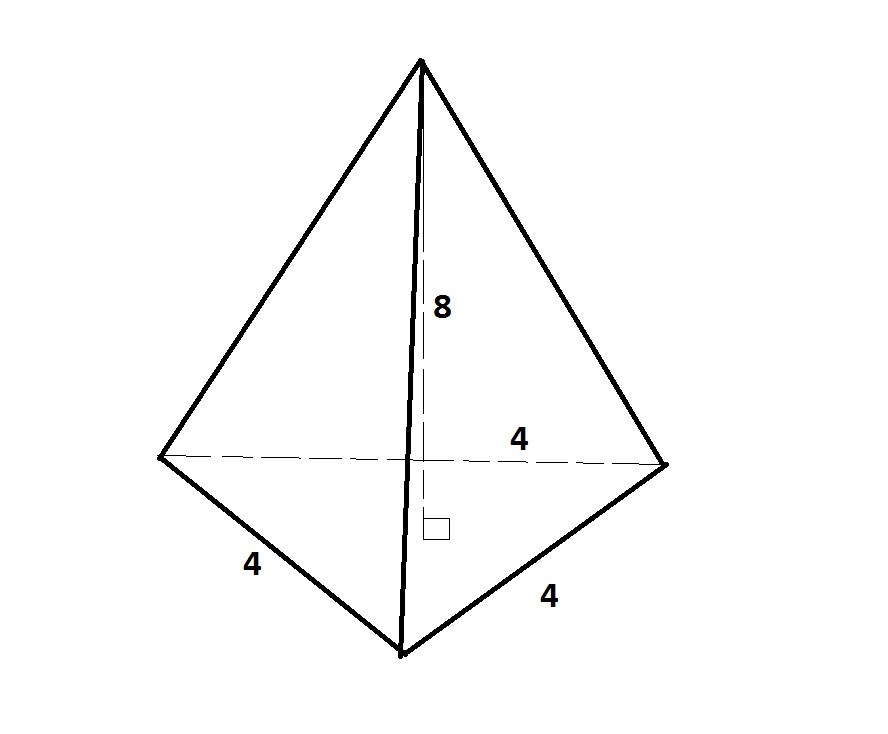Note: Figure NOT drawn to scale.

Give the volume (nearest tenth) of the above triangular pyramid.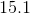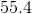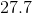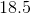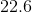Explanation:

The height of the pyramid is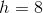. The base is an equilateral triangle with sidelength 4, so its area can be calculated as follows:The volume of a pyramid can be calculated using the fomula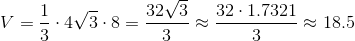### Example Question #82 : Solid Geometry

A regular tetrahedron has an edge length of. What is its volume?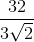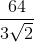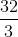Explanation:

The volume of a tetrahedron is found with the equation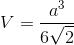, whererepresents the length of an edge of the tetrahedron.

Plug in 4 for the edge length and reduce as much as possible to find the answer: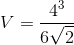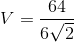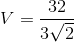The volume of the tetrahedron is.